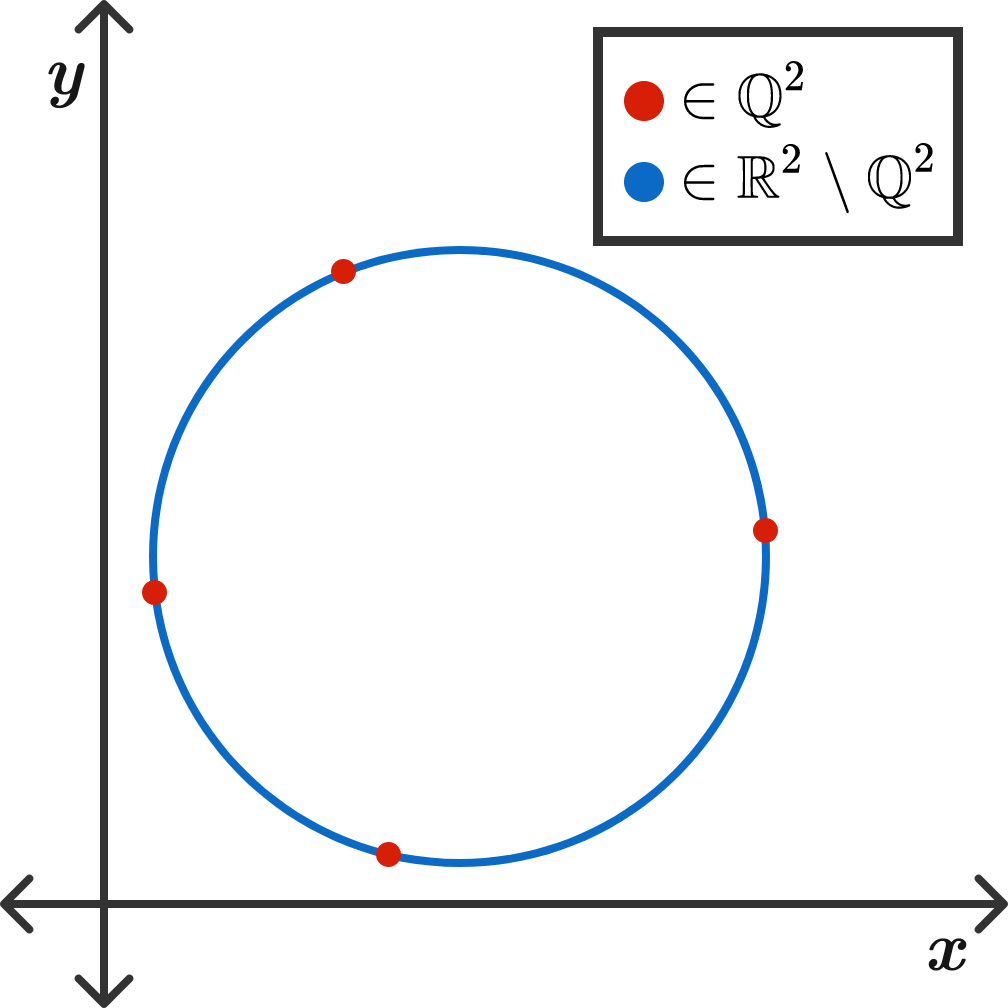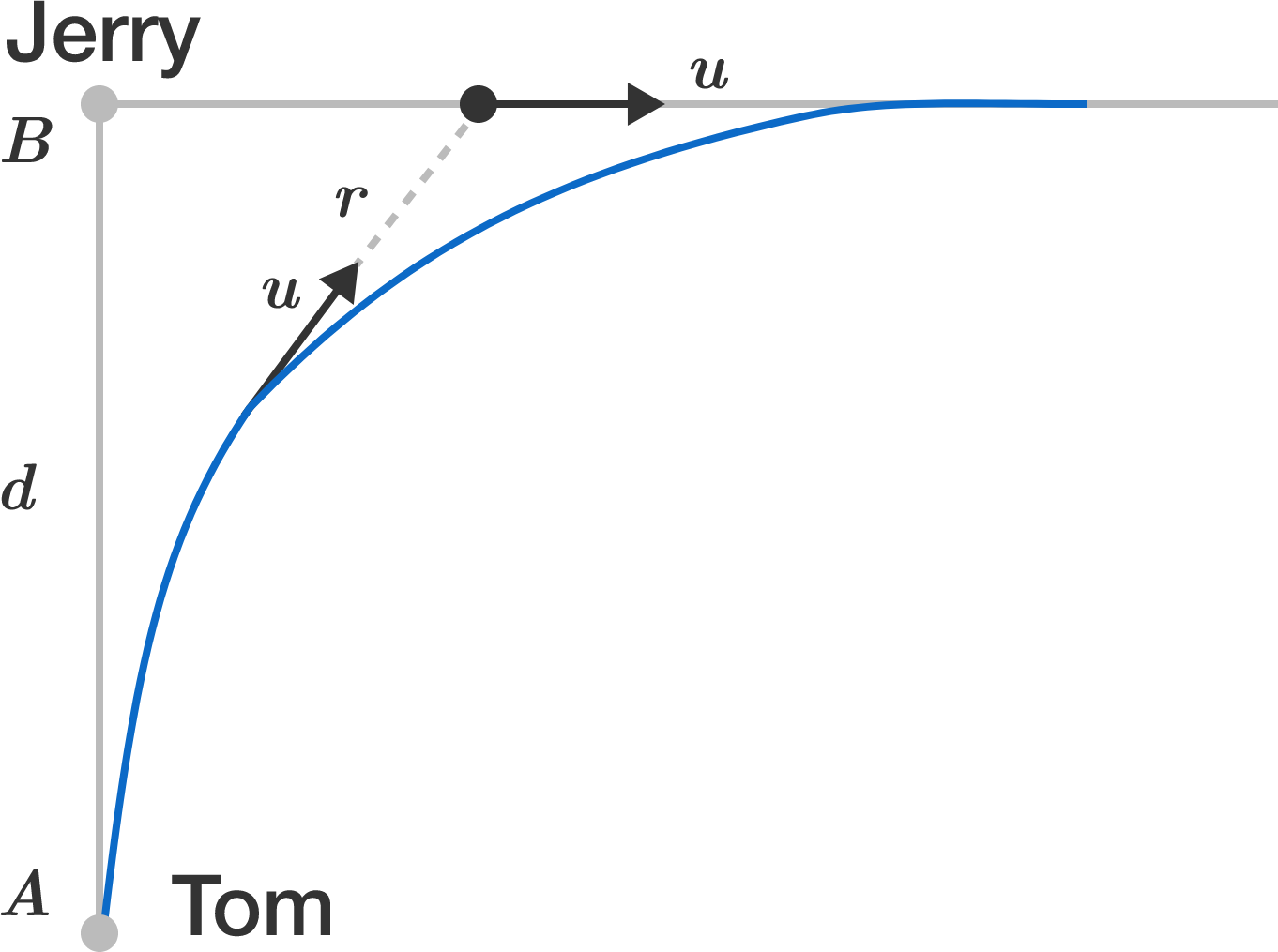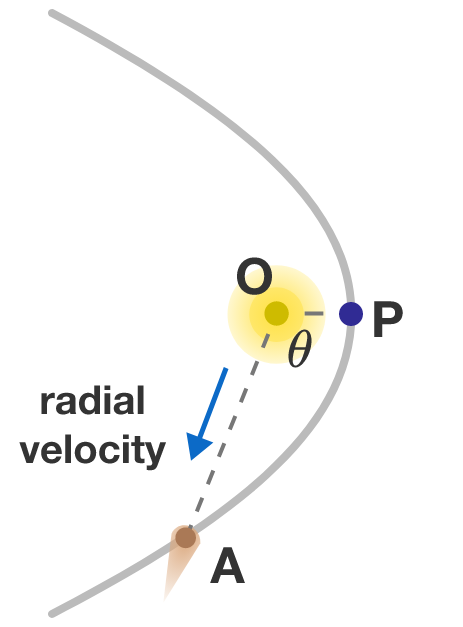# Problems of the Week

Contribute a problem

Four boats are on a large lake arranged so that they form the corners of a square of edge length $\ell = 1\text{ km}.$

At time zero, each boat starts moving with velocity $v_\textrm{boat} = \SI[per-mode=symbol]{10}{\kilo\meter\per\hour}$ such that its bow is always pointed directly toward its counterclockwise neighbor.

How long will the boats take to collide (in minutes)?Let $f(x)$ be a polynomial with integer coefficients such that

\begin{aligned} f(1) &= 1 \\ f(2) &= 8 \\ f(3) &= 27 \\ f(5) &= 125 \\ f(6) &= 216 \\ f(7) &= 343. \end{aligned}

What is the minimum possible value of $|f(4)|?$

Inspiration.

Is there a circle in the Cartesian plane which passes through exactly 4 points with rational coordinates?Tom and Jerry both have equal top running speeds and are initially at points $A$ and $B,$ respectively, separated by a distance of $d$.

They both spot each other and immediately start running at their top speeds. Jerry runs on a straight line perpendicular to the line $AB$ and Tom runs in such a way that its velocity always points towards the current location of Jerry.

Let $r(t)$ denote the distance between Tom and Jerry at time $t$.

Find $\displaystyle \lim_{t \to \infty} r(t)$.A comet in the Oort cloud falls towards the Sun. As it picks up speed from the Sun's gravity, it accidentally picks up a very tiny extra velocity boost from a nearby object in the solar system, so that its trajectory is now a true parabola. It eventually whips around the Sun and begins to travel back out into the far reaches of space.Let $\theta$ be the angle $\angle POA,$ where point $O$ is the center of the Sun, point $P$ is where the comet has come closest to the Sun, and point $A$ is where it is on the trajectory outward.

The radial velocity of the comet relative to the Sun is the speed in which the comet is moving away from the Sun in the direction of the line $OA$. For what angle $\theta$ is this radial velocity the greatest?


Note: Kepler orbits are commonly described by the polar equation $r(\theta)=\dfrac{a(1-e^2)}{1+e \cos(\theta)},$ where $a$ is the semi-major axis, and $e$ is the eccentricity. For a circle, $e=0;$ for an ellipse, $0 for a parabola, $e=1;$ for a hyperbola, $e>1$. Also, you may want to look up Kepler's laws and vis-viva equation.